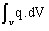# Describing sources

## Introduction

We distinguish three types of sources:

• an imposed value of heat sources (q0)
• an imposed value of heat flux density (φ0)
• an imposed value of temperature (T0)

The describing of these three types of sources in Flux software is detailed in the following blocks.

## Imposed heat sources

It is possible to impose a density of heat sources (q0) on a region by: using the material regions of conducting type (as presented previously): the volume density of heat sources is then one of the data entered during the definition of the conducting region.

The power volume density of the heat sources is one of the input data during the definition of the conducting regions. The power volume density of the heat sources represents the q term in the thermal equations of steady state and transient thermal applications (see § Solved equation).

The power of a heat source is the volume integral of q over the volume of the source ().

The power volume density of the heat sources can be calculated with the electric conduction or magneto-harmonic applications (Joule losses).

## Imposed temperature

It is possible to impose a value of temperature (T0) on a region by using the regions known as non-material of the type: imposed temperature

## Imposed heat flux density

It is possible to impose a value of heat flux density (φ0) on a region by using the regions known as non-material of the type: heat exchange surface